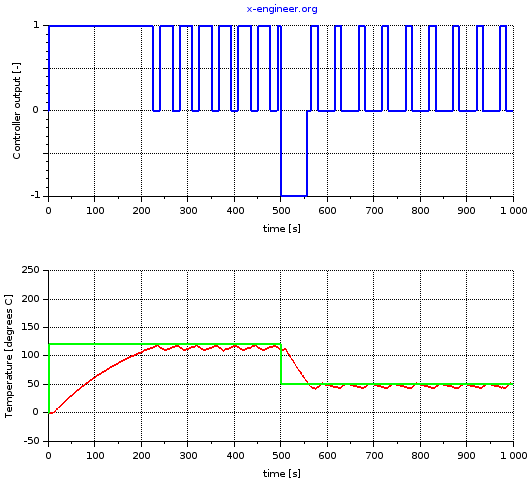# How to integrate C code in Xcos

C code is widely used in engineering applications, the vast majority of electronic control devices have software written in C programming language.

In all software development processes, every parts of the code must be fully testes before reaching production stage. Also, there are projects which need to integrate legacy C code with a modern simulation environment, like Xcos. Last but not least, having a controller written directly in C code is much more efficient from the resource usage point of view (memory and run time). In all these use cases, the controls (software) engineers will have to integrate C code with Xcos block diagrams.

As example, how to integrate C code with Xcos block diagrams, we’ll design an On-Off controller with deadband (DB) for the temperature control of an industrial oven. To understand how an On-Off controller works and also the system setup, read the article On-off control system.

As stated, the purpose of the controller is to regulate the temperature of the oven around a temperature setpoint (target). The output of controller will be discrete, with the following states:

• HOLD: in this state, the temperature is maintained around the setpoint
• HEAT: in this state, the temperature is increased towards the setpoint
• COOL: in this state, the temperature is decreased towards the setpoint

When the temperature difference (error) between the setpoint and the actual oven temperature is bigger that half of the deadband, the controller should start to HEAT the oven. When the error (temperature difference) is within the deadband, the controller will be in HOLD state, neither heating nor cooling being required. When the error is negative, less than half of the deadband, the controller should COOL the oven.

The Xcos block diagram for the temperature control system is depicted in image below.

The temperature setpoint is time dependant and is defined using an Interpolation block, where the x-axis is time and y-axis the temperature. The temperature error is calculated as the difference between the setpoint and the actual temperature of the oven (plant). The controller has the temperature error as input and outputs a 3-state control signal.

The plant (oven) is modelled as a first order transfer function with a transport delay (10 s).

The controller is a hybrid between Xcos blocks and custom C code. Before diving into the details of the controller, let’s design a state machine which describes how our controller works. From the state diagram above we know that the controller has 3 states: HOLD, HEAT and COOL, each state being encoded by an integer number.

The transitions between the states are function of the temperature difference (error) and the deadband. The controller will output a control signal, which has a specific value in each state.

An example of C code for the state machine is:

```switch (stateIN){
case 0:
// OFF
if (error>DB/2) {
stateOUT=1;}
else if (error<-DB/2) {
stateOUT=2;}
else {
control=0;}
case 1:
// HEAT
if (error<=DB/2) {
stateOUT=0;}
else { control=1;}
case 2:
// COOL if (error>=-DB/2) {
stateOUT=0;}
else {
control=-1;}
}
```

`stateIN` is the current state of the controller, `stateOUT` being the state we want to go into, function of the temperature error.

A easy way of integrating this C code into Xcos is by using the `CBLOCK` block, from the User-Defined Functions palette.

When double-clicking on the CBLOCK we are given a user interface in which we have to define the number of inputs, outputs, parameters and name of C function. For our example we are going to have three inputs (error, deadband and input state) and two outputs (control signal and output state).

After the block parameters definition, a template of the C code is generated. Our bespoke code will need to be integrated into the template and link the local variables with the inputs and outputs of the `CBLOCK`.

```#include
#include
void ON_OFF_SM(flag,nevprt,t,xd,x,nx,z,nz,tvec,
ntvec,rpar,nrpar,ipar,nipar ,u1,nu1,y1,ny1)

double *t,xd[],x[],z[],tvec[];
int *flag,*nevprt,*nx,*nz,*ntvec,*nrpar,ipar[],*nipar,*nu1,*ny1;
double rpar[],u1[],y1[];
/* modify below this line */
{
double error, DB,control;
int stateIN, stateOUT;

DB = u1;
error = u1;
stateIN = (int)u1;

switch (stateIN){
case 0:
// OFF
if (error>DB/2) {
stateOUT=1;}
else if (error<-DB/2) {
stateOUT=2;}
else {
control=0;}
case 1:
// HEAT
if (error<=DB/2) {
stateOUT=0;}
else { control=1;}
case 2:
// COOL if (error>=-DB/2) {
stateOUT=0;}
else {
control=-1;}
}
y1=control;
y1=stateOUT;
}
```

The generic variable for all inputs is the array `u1[]` and the generic variable for all outputs is the array `y1[]`. By assigning the right index, we can link the C code variables with the Xcos environment. For example, the deadband is set to `10` °C in the Xcos environment and read into the C code as first input `DB = u1;`. The control signal is the first output to the Xcos environment and is linked with `y1 = control;`.

The default state of the controller, HOLD, is set by the initial value (`0`) of the Unit delay.

Running the simulation for `1000 s` will output the following graphical window:Image: On-off controller temperature output – Xcos (setpoint – green, actual – red)

The initial temperature of the oven is `0` °C. The first `500 s` of the simulation the target temperature is `120` °C. The controller will be in HEAT state and output value `1`. When the error becomes less or equal than `5` °C, the controller switches to HOLD state, and outputs `0`. In this state the oven temperature decreases slowly and if the error becomes greater than `5` °C, the controller enters in HEAT state.

After `500 s`, the target temperature switches to `50` °C. In order to drop the temperature faster, the controller goes into COOL state and outputs `-1`. When the target temperatures is reached, within the deadband, the controller switches between HEAT and HOLD states.

The purpose of this article was to explain how to integrate a bespoke C code function into the Xcos environment. The same methodology can be used to integrate different types of controllers.